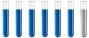## Explanation required for 'AmmoniaConcentration' package in 'R'

Chemistry and homework help forum.

Organic Chemistry, Analytical Chemistry, Biochemistry, Physical Chemistry, Computational Chemistry, Theoretical Chemistry, High School Chemistry, Colledge Chemistry and University Chemistry Forum.

Share your chemistry ideas, discuss chemical problems, ask for help with scientific chemistry questions, inspire others by your chemistry vision!

Please feel free to start a scientific chemistry discussion here!

Discuss chemistry homework problems with experts!

Ask for help with chemical questions and help others with your chemistry knowledge!

Moderators: Xen, expert, ChenBeier

Dhamnekar Winod
Sr. Staff MemberPosts: 243
Joined: Sat Nov 21, 2020 10:14 am
Location: Mumbai[Bombay] and Mumbai Suburb,, Maharashtra State,India

### Explanation required for 'AmmoniaConcentration' package in 'R'

Description of 'AmmoniaConcentration' package in 'R'.

The total ammonia in aqueous solution is present in two chemical species: un-ionized ammonia,
NH3 , and the ionized form, NH4 +. The NH3 species is the one more toxic for aquatic organisms,
but current analytical methods do not permit measurement of NH3, and NH4 + separately. The
concentration of each chemical species in the total ammonia is dependent of a number of factors,
with the pH and the temperature being the most important.
The equation presented by Emerson et al. (1975) permits the calculation of the NH3 fraction from
the total ammonia measured in freshwater, as long as you also have the pH and temperature data
from the sample. The calculus first calculates the pKa, which is the ionization constant of the
ammonium ion. To calculate the pKa value of the sample, we use the equation:
pKa = 0.09018 + 2729.92/T
where T is the temperature in Kelvin.
To calculate the fraction of NH3 , we use the equation:
f = 1/(10(pKa − pH) + 1)
Note: the equation for pKa is invalid outside the temperature range of 0-50 C (273-323 Kelvin),
because is the range where the pKa values used to make the equation were obtained empirically.

Usage
ammonia(total_ammonia, temperature, ph, type_of_temperature)
Arguments
total_ammonia:- Total ammonia (NH3 + NH4) in the aqueous solution
temperature:- Temperature of the aqueous solution
ph:- pH of the aqueous solution
type_of_temperature:-Unit of measure of temperature, "K" for Kelvin, "C" for Celsius and "F" for Fahrenheit
Value
The function returns a list with all steps of the calculation. pka: the immunization constant; pka_ph:
the difference between pka and the informed pH; ten_pka_ph: 10 to the power of pka_ph; nh3: the
fraction of NH3; nh3_mgL: the concentration of un-ionized ammonia in the acqueous solution

We know that,

pKa = -logKa, pH = pKa + log (A-)/HA, Ka= (H+)(A-)/HA

Then,

How did the author derive the highlighted equations in the above description?

How to interpret the following answers using "AmmoniaConcentration" package in 'R' ?

> library("AmmoniaConcentration")
> ammonia(5.14, 294.4, 6.9, "K")
$pka  9.363006$pka_ph
 2.463006

$ten_pka_ph  290.4063$nh3
 0.003431634

$nh3_mgL  0.0176386 > ammonia(0.98, 27.7, 8.05, "C")$pka
 9.16873

$pka_ph  1.11873$ten_pka_ph
 13.14408

$nh3  0.07070098$nh3_mgL
 0.06928696
Any science consists of the following process. 1) See 2)Hear 3)Smell,if needed 4)Taste, if needed 5)Think 6)Understand 7)Inference 8)Take decision [Believe or disbelieve, useful or useless, true or false, cause or effect, any other criteria]